# Gergely Kalapos

## Struct layout in C# - .NET Concept of the Week - Episode 13

Posted on May 11, 2018

In this episode I talk about struct layout in C#. You will learn how C# structs are represented in memory and you will also learn how you can influence this with the StructLayoutAttribute.

## The written form of the video:

Let’s start with the usual warning, when it comes to low level things:

Make sure you do not overuse or overthink this stuff. If you work on a typical business application then what we talk about here doesn’t really matter, since the potential performance benefit is negligible. On the other hand, if you have code with value types that are potentially accessed let’s say more than 1 million times a second, then this stuff can matter.

All right, let’s move on!

Not long ago, Sam tweeted this very interesting stuff regarding structs in C#.

We have two structs here, both of them contain 3-byte fields and 2 double fields.

The only difference is the ordering of the fields. As you can see in the first case we have one-byte field, then a double field, then a byte field, and so on, and in the second case we have the two doubles first and then the three-byte fields.

And the interesting thing is that the size of the two structs is different: the first one is 40 bytes, the second one is only 24.

### So, first obvious question: What is the reason for this?

In C# the compiler by default makes sure that the fields of value types are stored in the same order as they are defined in the C# code. Additionally, the fields of a type instance are aligned by using specific rules.

These rules can be found on MSDN.

• So first: "the alignment of the type is the size of its largest element or the specified packing size, whichever is smaller". Now let’s ignore the packing size, we will talk about that later. In our case the largest type was a double, which is 8 bytes, so our struct will be aligned to 8 bytes.
• Second: "each field must align with fields of its own size, or the alignment of the type, whichever is smaller."
So, in case of an 8-byte double field this means that a double field can only start at position 0, 8, 16, 24, and so on.
• And last but not least: "padding is added between fields to satisfy the alignment requirements."

So what by default happens is that the compiler makes sure that every field from structs is aligned according to the rules that we just discussed. Now when I say "by default" I mean that we create a struct without any additional attribute.

So, this one is the first struct:

``````struct Struct1
{
public byte b1;
public double d1;
public byte b2;
public double d2;
public byte b3;
}``````

The first field is a byte, which -well- is 1 byte big and it will be placed at position 0.

The second field is a double, which is 8 bytes. Now as we saw, by default the compiler makes sure that doubles are aligned at multiple of 8 -this is the second rule-, so in this case it places the second field to position 8. Between the end of the byte field and the beginning of the double field we have padding, so those bits aren’t used. Then we have the 3. field, which is 1 byte again, we place it to position 16, then we have a double, and here we need padding again, so we place it to position 24, and then we have our last byte field, which will start at position 32. Now structs must be aligned with the largest member type -remember, this was the first rule-, which is in this case the 8-byte big double type, and 32 + 8 is 40, and therefore the size of this struct is 40.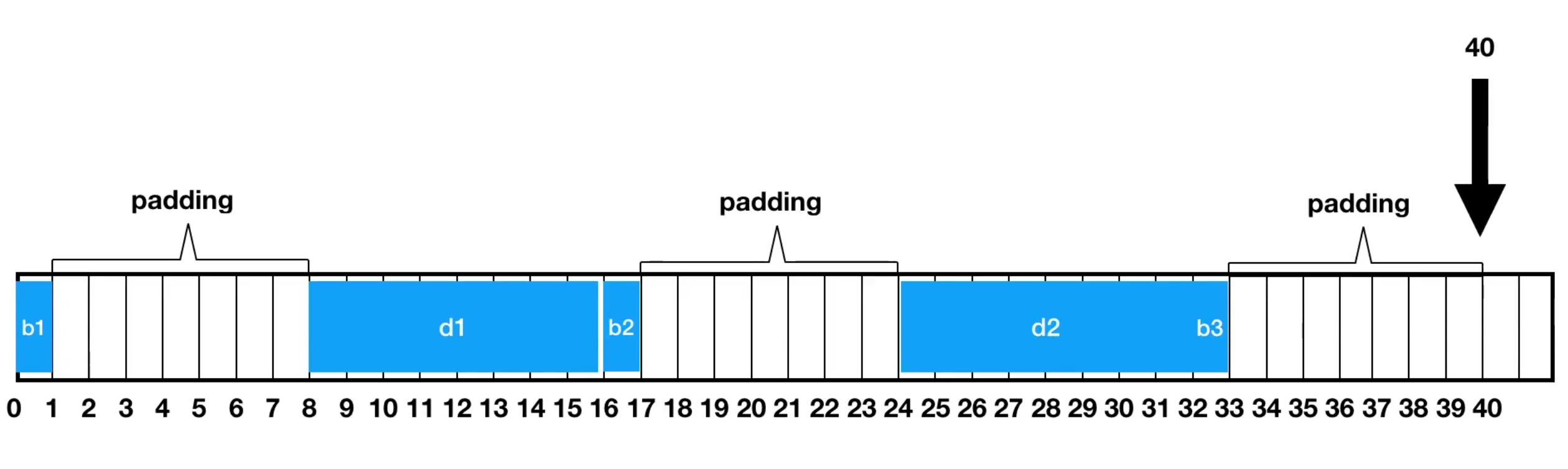Now let’s do the same with the second struct!

``````struct Struct2
{
public double d1;
public double d2;
public byte b1;
public byte b2;
public byte b3;
}``````

So, we place the first double at position 8, and its 8 byte long, so we won’t need padding here. Then we have another double field, which starts at position 8. Then we have our first byte field which we place at position 16 and it will take 1-byte space. Then we have our next byte field, which is 1 byte again, so it still fits into this bucket -remember the second rule, a 1-byte field is aligned to 1 byte-, so we place it right next to the first byte field. Then we have the 3. byte field and we do the same with it. Until this point we used 19 bytes, but again, the struct must be aligned with the largest member, which is 8, so 16 + 8 equals to 24, and this is exactly what the sizeof operator told us.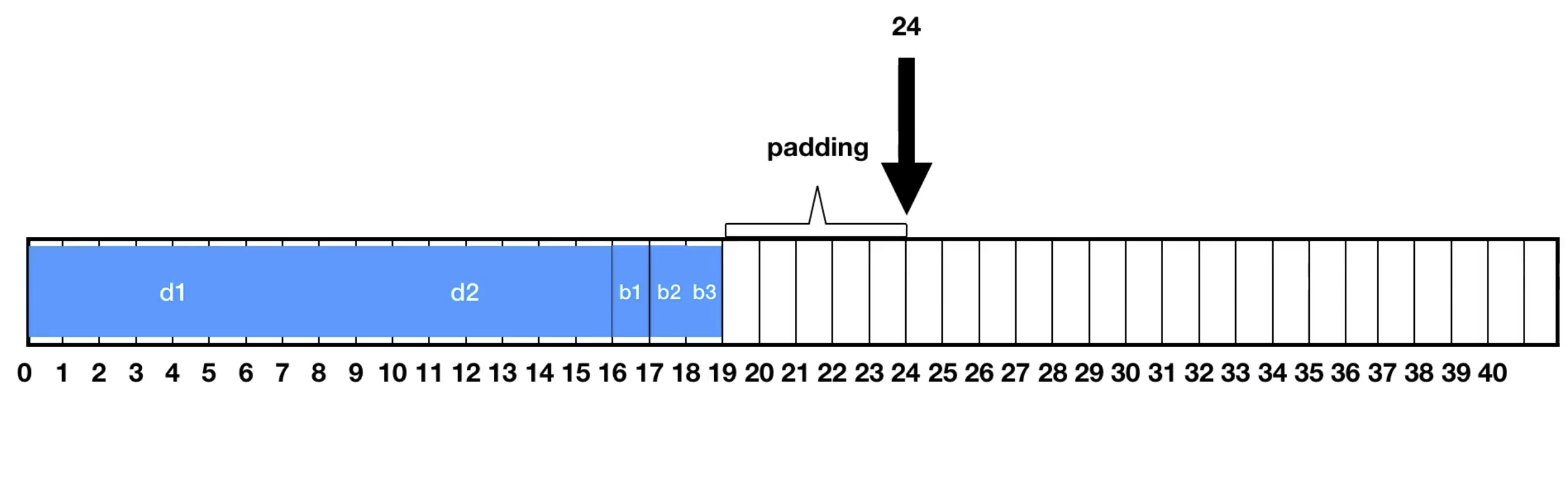With that we now understand that by default the fields in a C# struct are stored sequentially and we also know the rules on how the fields are aligned.

We saw that by changing the order of the fields we were able to get a smaller struct.

### The next question is: why doesn’t the compiler automatically do this reordering for us?

The answer is that the developers of the C# compiler decided to use sequential layout for structs by default and it has a historical reason: in the early days of .NET it was assumed that structs will be commonly used when interoperating with unmanaged code, and for that to work, the fields must stay in order.

And by the way we can even see this if we look at the compiled code: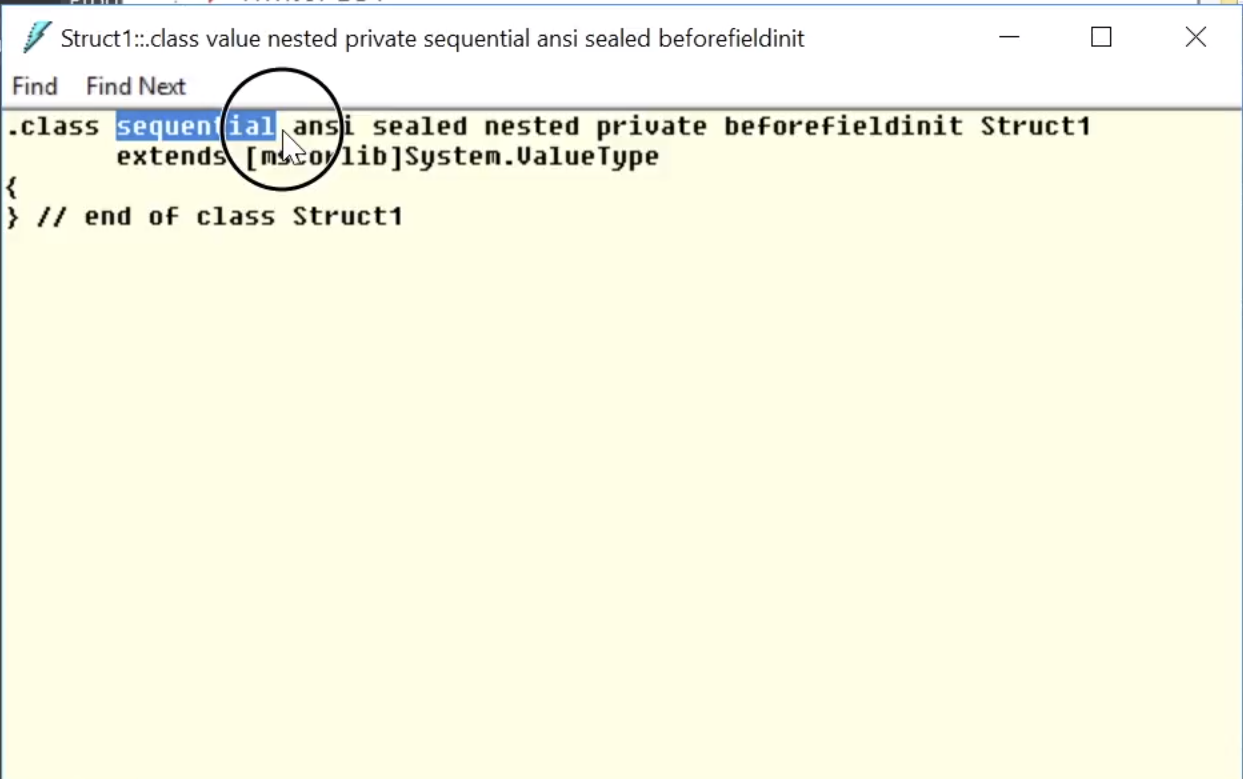I simply opened the dll with our struct in ILDasm. We have "class sequential", a bunch of other things and then "extends System.ValueType" here. The important keyword for us here is "sequential", this means that the CLR makes sure that the fields aren’t reordered.

All right, next question:

### If I know that my C# structs will only be used in managed code, can I change this behavior and let the compiler reorder the fields to minimize padding?

Yes! For that we can use the StructLayout attribute. It offers 3 options:

• Sequential - this is the default, and this is exactly what we saw before
• Auto
• Explicit.

### Let’s focus on auto struct layout!

By setting StructLayout to LayoutKind.Auto we enable the compiler to reorder the fields.

``````[StructLayout(LayoutKind.Auto)]
struct Struct1
{
public byte b1;
public double d1;
public byte b2;
public double d2;
public byte b3;
}
``````
So, we still have the alignment rules with padding, but as we saw in the original example there are cases where by reordering fields we can have less padding -or gaps- between our fields. Now with Auto layout you cannot pass this struct to unmanaged native code, on the other hand as long as you only use it in managed code you potentially save memory with auto layout without thinking about the order of your fields.

I compiled this code and I open it in ILDasm again.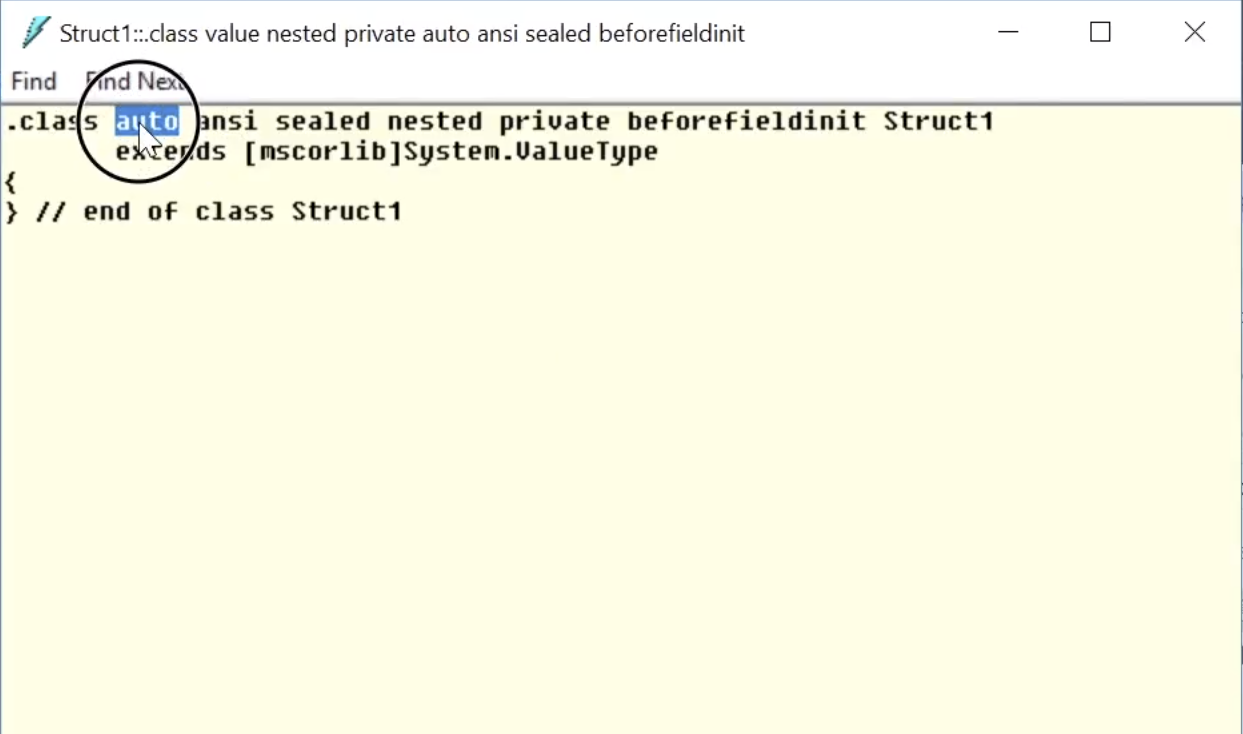And as you can see this time we have ".class private auto" here instead of "sequential". So, this auto flag in the IL code tells the CLR to enable field reordering in this struct.

We also have a class here and as you can see this also has this auto flag: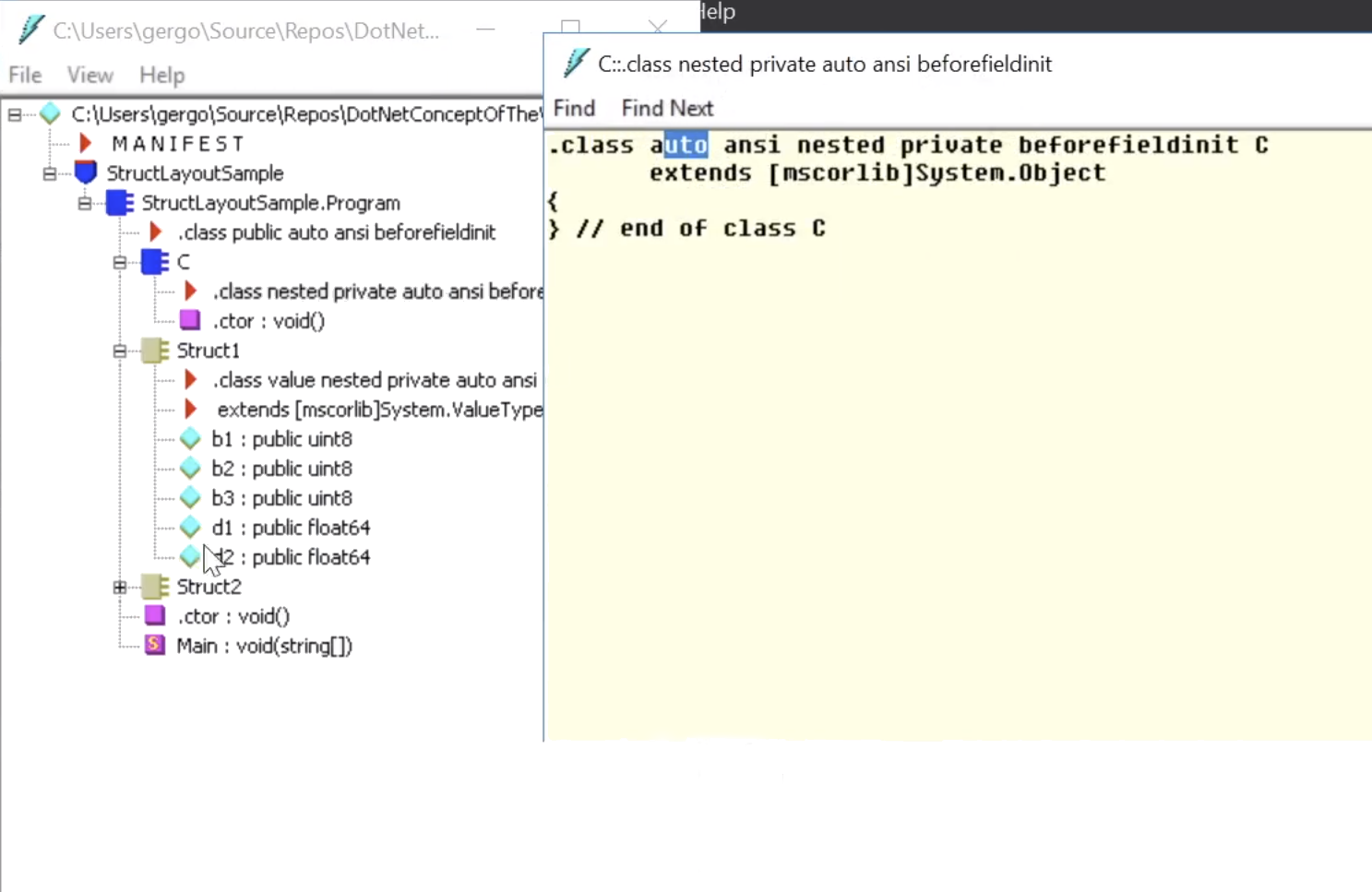So, in the C# compiler the alignment default for classes is auto and for structs is sequential.

All right, now next question:

### There was this packing size in the alignment rules, what is that?

On the StructLayoutAttribute you can define a packing size, here is how you can do it:

``````[StructLayout(LayoutKind.Sequential, Pack = 1)]
struct StructWithPack1
{
public byte b1;
public double d1;
}``````

So, the first rule says that "the alignment of the type is the size of its largest element or the specified packing size, whichever is smaller."
Now if we set this to 1 then it means that the type itself will be aligned to 1 bytes, which means that we won’t need padding after the last field.
The second rule says that "each field must align with fields of its own size -we already saw that- or the alignment of the type, whichever is smaller". If the pack size is 1 then the alignment of the type is 1, so each field is also aligned with 1 byte.

Let’s see an example:

``````[StructLayout(LayoutKind.Sequential, Pack = 1)]
struct Struct1
{
public byte b1;
public double d1;
public double d2;
public byte b2;
public byte b3;
}
``````
I have a 3. struct here with packsize 1 -it has the same fields as our previous structs-, the first item is a byte, it goes to position 0, and its size is 1. Then we have a double field, which is 8 byte, now remember the second rule, its aligned either to its size (which is 8) or to the pack size, whichever is smaller, in this case packsize is 1, so it’s aligned to 1 byte, therefore we put it to position 1, then we have a double again, same story, we put it to position 9, then we have two bytes, those are again aligned to 1 byte, so they go to position 17 and 18.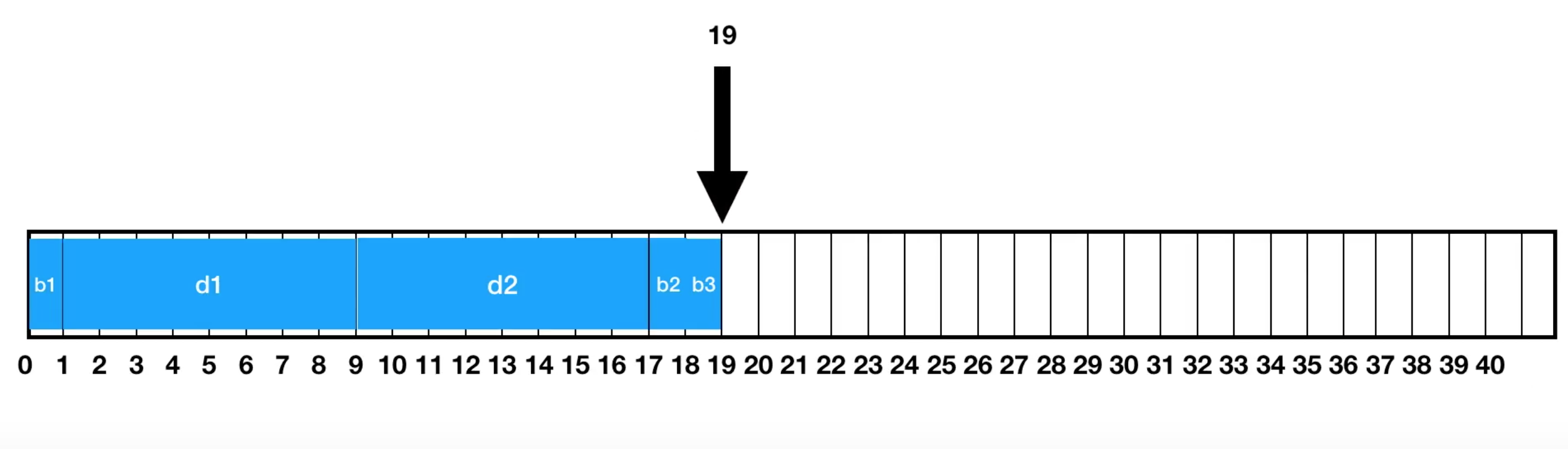And that’s it, so the size of this struct is 19.

And as you can see, once I run this, it really says 19 (the first line is the size of our new struct with Pack=1):### What can be the problem with this? And why isn’t 1 the default, since with that we can completely avoid padding?

Well, the problem with this is that CPUs need specific alignment to be able to access fields efficiently. If you set the pack size to for example 1 then you can end up with fields on a memory boundary that is very inefficient to access. So, before you change the packsize in production code I’d suggest measuring the difference first because you can easily end up with smaller structs and still slower runtime.

### Now for completeness let’s also talk about Explicit alignment!

We talked about sequential and auto StuctLayout, the third option is explicit layout. If you set the StructLayout attribute to explicit layout then you can use the FieldOffset attributes to tell the CLR the offset of the fields within a struct.

This also came up in the twitter discussion, and one cool use case can be to implement a union with this.

``````[StructLayout(LayoutKind.Explicit)]
struct Union
{
[FieldOffset(0)]
public byte b;
[FieldOffset(0)]
public int i;
[FieldOffset(0)]
public bool boolean;
}``````

So, we have 1 struct here with explicit layout. Now all 3 fields start at offset zero, so in this case this Union is either a byte, or an integer, or a Boolean, depending on how we use it.

Here is a little bit of sample code using it, so we can simply store an integer in it, and then use it, then we can interpret the integer as a Boolean, and so on:

``````Union u = new Union();
u.i = 42;
Console.WriteLine(u.boolean);
u.boolean = false;
int b = u.i``````

The important thing is its size: as you can see it’s only 4 bytes, and that is because the integer is 4-byte.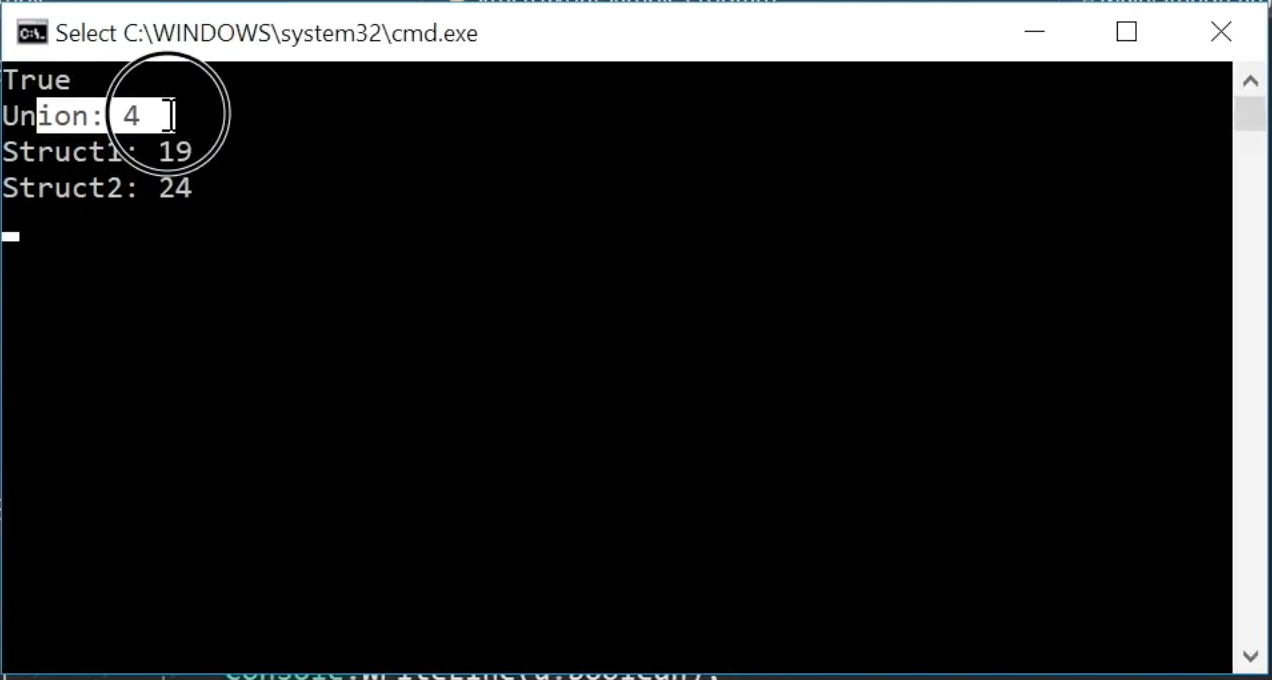With explicit layout we can create a struct with a size that is smaller than the sum of the size of its fields.

Sam's tween (that inspired this video/post)

StructLayoutAttribute doc (Microsoft)
StructLayoutAttribute.Pack doc with the alignment rules (Microsoft)

SharpLab.io by @ashmind
Sergey Teplyakov - ObjectLayoutInspector

Sample code: on GitHub
Full playlist with other episodes: on YouTube

Music: bensound.com

;